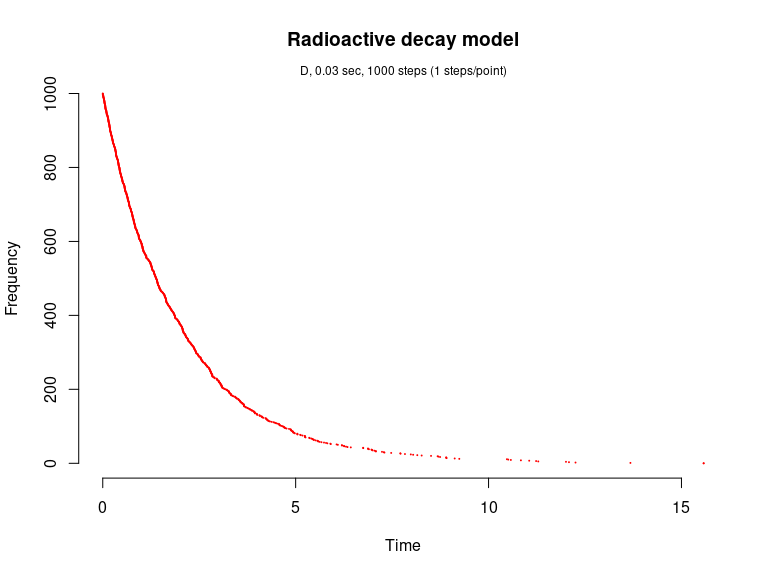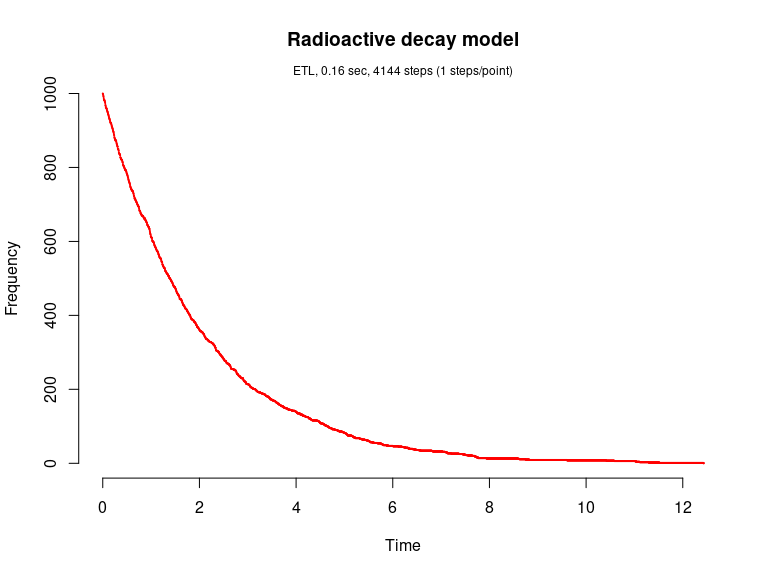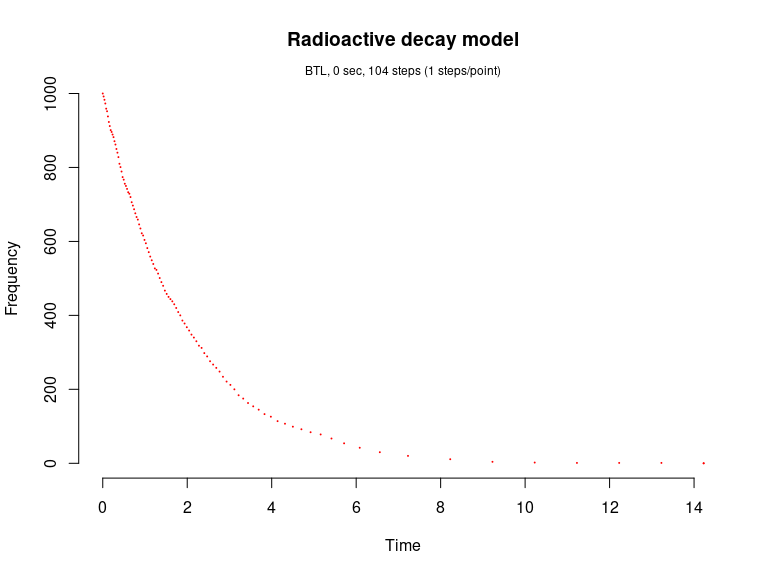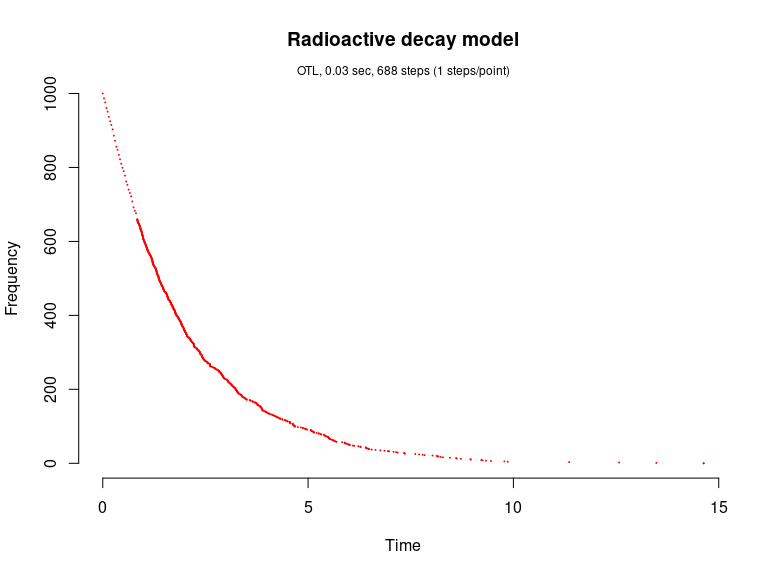# Radioactive decay model (Gillespie, 1977)

This model is also known as the irreversible isomerization reaction set. It consists of a single species and single reaction channels,

X --c--> 0

library(GillespieSSA)

Define parameters

parms <- c(k = 0.5)
tf <- 20                                       # Final time
simName <- "Radioactive decay model"

Define initial state vector

x0 <- c(N=1000)

Define state-change matrix

nu <- matrix(c(-1),nrow=1,byrow=TRUE)

Define propensity functions

a <- c("k*N")

Run simulations with the Direct method

set.seed(1)
out <- ssa(
x0 = x0,
a = a,
nu = nu,
parms = parms,
tf = tf,
method = ssa.d(),
simName = simName,
verbose = FALSE,
consoleInterval = 1
)
ssa.plot(out, show.title = TRUE, show.legend = FALSE)Run simulations with the Explict tau-leap method

set.seed(1)
out <- ssa(
x0 = x0,
a = a,
nu = nu,
parms = parms,
tf = tf,
method = ssa.etl(tau = .003),
simName = simName,
verbose = FALSE,
consoleInterval = 1
)
ssa.plot(out, show.title = TRUE, show.legend = FALSE)Run simulations with the Binomial tau-leap method

set.seed(1)
out <- ssa(
x0 = x0,
a = a,
nu = nu,
parms = parms,
tf = tf,
method = ssa.btl(),
simName = simName,
verbose = FALSE,
consoleInterval = 1
)
ssa.plot(out, show.title = TRUE, show.legend = FALSE)Run simulations with the Optimized tau-leap method

set.seed(1)
out <- ssa(
x0 = x0,
a = a,
nu = nu,
parms = parms,
tf = tf,
method = ssa.otl(),
simName = simName,
verbose = FALSE,
consoleInterval = 1
)
ssa.plot(out, show.title = TRUE, show.legend = FALSE)